Next: Problem Up: Outflow Previous: Outflow   Contents

## Magneto-driven Model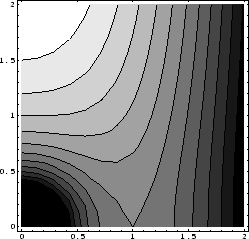To accelerate gas till supersonic speed, the magnetic force is considered to be an important player. Blandford & Payne (1982) have proposed the magneto-centrifugal wind model'' for the acceleration in two-dimensional axisymmetric configuration.

Figure 4.10 explains the magneto-centrifugal wind mechanism (Fig. 1 of Blandford & Payne 1982). Consider a disk under the gravity of a central star with mass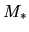. Assume that gas in the disk at the radius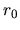rotates with a Kepler speed of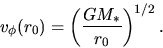(4.84)

Consider a gas parcel whose original position was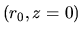. The effective potential for the particle rotating with an angular rotation speed of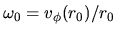is written as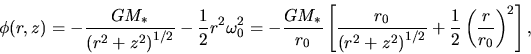(4.85)

where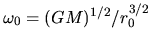. (Be careful that ordinary effective potential is calculated for constant angular momentum. However, this is for constant angular rotation speed.) The gas will co-rotate with the same angular rotation speed as the disk, if the magnetic field is sufficiently strong. Equation (4.85) corresponds the effective potential for the gas co-rotating with the disk at. Figure 4.10 plots the isopotential contour lines for the effective potential [eq.(4.85)]. The contour line passing the point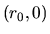represents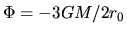. The angle between the isopotential line ofand the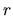-axis is equal to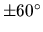(see problem below). Further, it can be obtained that the effective potential increases upwardly while it decreases with reaching the central star or radially outwardly.

This leads to an important conclusion that

1. If the angle between the magnetic field line and the-axis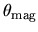is larger than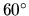(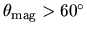), gas parcel moving along the field line must climb the effective potential. It needs extra energy to depart from the disk.
2. If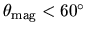, gas parcel moving along the field line slides down the effective potential. It can depart from the disk without extra energy.
Be careful that this is valid if the magnetic field is sufficiently strong. If the Kepler disk is threaded with the magnetic field lines with a shallow angle, and if the angular momentum is sufficiently transferred along the magnetic field line, in this case, the gas is accelerated by the extra centrifugal force and finally escapes from the potential well of the central star. This mechanism is called the magneto-centrifugal wind'' mechanism.

SubsectionsNext: Problem Up: Outflow Previous: Outflow   Contents
Kohji Tomisaka 2007-07-08